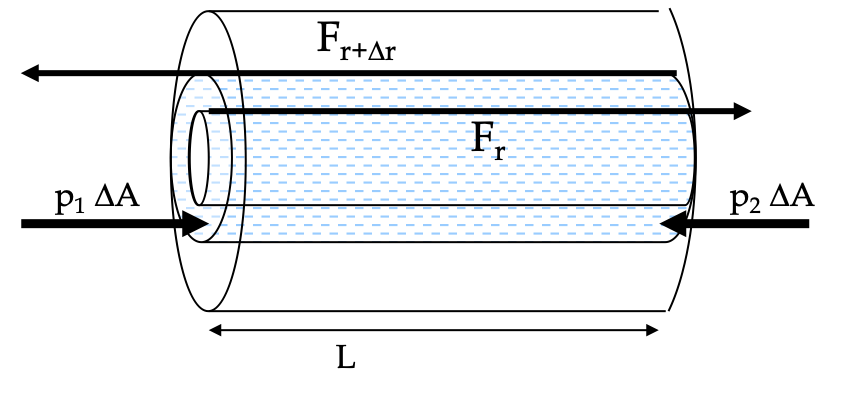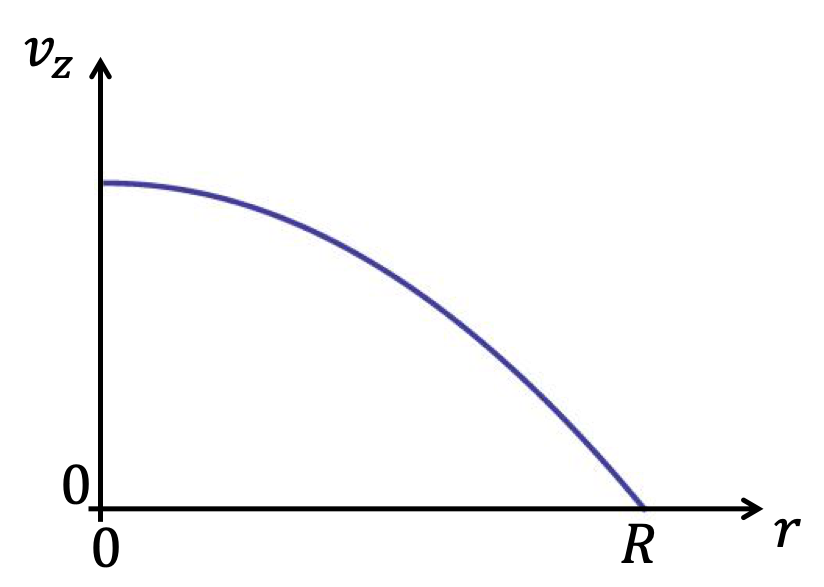## Section18.6Poiseuilli's Law

The French physician Jean Poiseuille studies the flow of fluids through uniform pipes experimentally and publised his results in 1840's. The results were theoretically derived by George Stokes in 1845 which made the conditions under which the law is applicable. We will present the derivation in the Calculus subsection below. We list here the conditions for the applicability of Poiseuilli's Law before we state the law.

1. Fluid is incompressible,
2. Fluid is Newtonian, meaning, the shear stress is linearly related to shear strain,
3. The length of the tube is much longer than its radius,
4. Fluid is not accelerating, and
5. Effects of gravity on flow are ignored.

The law states that the volume flow rate of the fluid flow $Q$ or $\Delta V/\Delta t$ in a tube of uniform circular cross-section is directly proportional to the pressure differnece $\Delta p$ across the tube and viscous resistance to the flow $\mathcal{R}\text{.}$

\begin{equation} Q\equiv \dfrac{\Delta V}{\Delta t} = \dfrac{\Delta p}{\mathcal{R}}.\label{eq-poiseuillis-law-1}\tag{18.6.1} \end{equation}

Poiseuille found by experiments that the resistance $\mathcal{R}$ is directly proportional to the length $L$ of the tube and inversely proportional to fourth power of the radius $R$ of the tube as long as $R \lt\lt L\text{.}$ Furthermore, the resistance $mathcal{R}$ is also proportional to the viscosity $\eta\text{.}$

\begin{equation} \mathcal{R} =\dfrac{8}{\pi} \frac{\eta L}{R^4}.\tag{18.6.2} \end{equation}

The surprising part of this resistance is the fourth power dependence on the viscous flow resistance. Putting $\mathcal{R}$ in Eq. (18.6.1) we get the following expression of Poiseuille's law for the pressure drop expected from the higher pressure end of the tube to the lower pressure end.

\begin{equation} \Delta p = \mathcal{R}Q = \dfrac{8}{\pi} \frac{\eta Q L}{R^4}.\label{eq-poiseuillis-law-dp}\tag{18.6.3} \end{equation}

Note the very strong dependence of the resistance on the radius of the pipe; if the radius is halved, then the resitance becomes four times, which means you would need four times as much pressure difference to maintain the same volume flow rate, or if your pressure difference is maintained at the same level, then the flow rate will drop to quarter of its value.

The Poiseuille's law is useful in the study of flow of blood in the body and other engineering applications.

One liter of blood flows per day through a narrow vein of length $5\text{ cm}$ which has a pressure difference of $0.5\text{ atm}$ across its length. What is the diameter of the vein?

Data: $\eta_\text{blood} = 2.7\text{ cP}\text{.}$

Hint

Use Poiseuille's law. Also, make sure you convert everything in the same system of units.

$224\, \mu\text{m}$

Solution

The Poiseuille's law says that

\begin{equation*} Q = \frac{\pi R^4}{8\eta L} \Delta p. \end{equation*}

Therefore,

\begin{equation*} R = \left( \frac{8\eta L}{\pi \Delta p}\ Q\right )^{1/4}. \end{equation*}

In this problem, various given quantities should be first converted into the same system of units. We will convert them into standard SI system.

\begin{align*} \amp Q = 1 \frac{\text{L}}{\text{Day}} = \frac{10^{-3}\ \text{m}^3}{24\times 3600\ \text{s}} = 0.01157 \ \text{m}^3\text{s}\\ \amp \Delta p = 0.5\ \text{atm} = 2.53\times 10^{4}\ \text{Pa} \\ \amp L = 0.05\ \text{m} \\ \amp \eta = 2.7\ \text{cP} = 2.7\times 10^{-3}\ \text{Pa.s} \end{align*}

Therefore, $R = 112\, \mu\text{m}\text{.}$ The diameter of the vein would be $224\, \mu\text{m}\text{.}$

An intravenous (IV) system is supplying saline solution to a patient at the rate of $750\text{ mL}$ in $180\text{ minutes}$ using a needle of inner radius $0.15\text{ mm}$ and length $2.54\text{ cm}\text{.}$ The pressure in the vein into which saline from the needle tip will enter is $1\text {atm} + 10\text{mm Hg}\text{.}$ Suppose the viscosity of saline solution is $1.002\text{ cP}\text{.}$

(a) What is the flow rate in $\text{m}^3\text{/sec}\text{?}$

(b) What is the pressure at the back part of the needle in units of $\text{mm Hg} \text{?}$

(c) What will be the pressure needed at the back part of the needle in units of $\text{ mm Hg}$ for the same flow rate if a thicker needle of radius $0.1475\text{ mm}$ is used?

Hint

(a) Use $V/\Delta t\text{.}$ (b) Use Poiseuille's law. (c) Use rescaling argument rather than a detailed calculation.

(a) $6.94\times 10^{-8}\text{ m}^3\text{/s} \text{,}$ (b) $836\text{ mm Hg} \text{,}$ (c) $894\text{ mm Hg} \text{.}$

Solution 1 (a)
\begin{equation*} \dfrac{ 750\text{ mL} }{ 180\text{ minutes} } = \dfrac{ 750\times 10^{-6} }{ 180 \times 60} = 6.94\times 10^{-8}\text{ m}^3\text{/s}. \end{equation*}
Solution 2 (b)

We convert units to SI, and then to $\text{mm Hg}$ from $\text{Pa}\text{.}$

\begin{align*} \amp R = 0.15\text{ mm}= 1.5\times 10^{-4} \text{ m}, \\ \amp L = 2.54\text{ cm}= 2.54\times 10^{-2} \text{ m}, \\ \amp p_{\text{vein}} = 1\text {atm} + 10\text{mm Hg} = 1.026 \times 10^{5}\text{ Pa}, \\ \amp \eta = 1.002\text{ cP} = 1.002 \times 10^{-3}\text{ Pa.s}, \end{align*}

Therefore,

\begin{align*} \Delta p \amp = \dfrac{8}{\pi} \frac{\eta L}{R^4} \\ \amp = \dfrac{8}{\pi} \frac{1.002 \times 10^{-3} \times 2.54\times 10^{-2}}{ (1.5\times 10^{-4})^4} \\ \amp = 8885\text{ Pa} \end{align*}

This gives pressure at the back end of the needle

\begin{equation*} p_{\text{high}} = p_{\text{vein}} + \Delta p = 1.026 \times 10^{5} + 8885 = 111485\text{ Pa}. \end{equation*}

We convert this to $\text{mm Hg}\text{.}$

\begin{equation*} 111485\text{ Pa} = 111485\times \dfrac{760}{1.013\times 10^{5}} = 836\text{ mm Hg}. \end{equation*}
Solution 3 (c)

We do not need to do a fresh calculation since we have same $Q\text{,}$ $L\text{,}$ and $\eta \text{.}$ We just rescale $\Delta p\text{.}$

\begin{equation*} \Delta p = 836\text{ mm Hg} \times \left( \dfrac{0.15\text{ mm}}{0.1475\text{ mm} }\right)^4 = 894\text{ mm Hg}. \end{equation*}

A blood clot in an artery essentially decreases the radius of the artery. Suppose a blood clot in a artery has reduced the blood flow to 85% of its normal flow rate and the pressure differece increased to 120% of the normal. Without much calculation, find the ratio of the current radius to the original.

Hint

Divide formulas for one situaion by another and cancel out the common factors.

90%

Solution

Lets use subscripts 1 for normal and 2 for clotted situaiton. From the pressure difference ratio and the flow rate ratio, we get the ratio of resitances in the two situations as

\begin{equation*} \dfrac{\mathcal{R}_2}{\mathcal{R}_1} = 1.20 \times \dfrac{1}{0.8} = 1.5. \end{equation*}

Now only thing different in resistance formulas are the radii. Therefore,

\begin{equation*} \left( \dfrac{R_1}{R_2} \right)^4 = 1.5, \end{equation*}

which gives

\begin{equation*} \dfrac{R_2}{R_1} = 1.5^{-1/4} = 0.90. \end{equation*}

That is, the new radius is 90% of the original.

### Subsection18.6.1(Calculus) Velocity Profile in a Flow Through a Cylidrical Pipe

Consider a viscous fluid flow at constant velocity through a straight cylindrical pipe of radius $R$ placed horizontally. Let there be a difference of pressure $\Delta p$ maintained across its ends separated by a distance $L\text{.}$

We assume that the velocity is such that we can assume a laminar flow. The layers will be in the shape of concentric cylindrical shells with the fluid at the center moving fastest while the fluid next to the non-moving wall is at rest. We would like to find the velocity profile, i.e., velocity as a function of the distance from the center.

Let us look at the fluid in a cylindrical shell of inner radius $r$ and outer radius $r+\Delta r$ about the central axis. The cylindrical shell is taken to be thin enough that we can assume the fluid in the shell to move with the same velocity $v_z\text{.}$The fluid just outside the shell moves at a lower velocity and therefore has a retarding effect on the cylindrical shell under consideration, while the fluid just inside moves at a higher velocity, and therefore tends to accelerate the fluid in the shell. Their difference is balanced by the force due to the pressure difference $\Delta p =p_1 -p_2$ of the pressures at the two ends.

Let the flow be in the direction of positive $z$ axis and the cross-section in the $xy$-plane. Thne, we have the following force balance equation on the shell.

\begin{equation} \Delta p \times 2\pi r \Delta r = F_z \left( r+\Delta r \right)- F_z \left( r \right),\label{eq-velocity-flow-profile-force-balance-equation-1}\tag{18.6.4} \end{equation}

where $F_z$'s are the viscous forces.

\begin{align*} \amp F_z (r) = 2\pi \eta L r\frac{dv_z}{dr}, \\ \amp F_z (r + \Delta r) =2\pi \eta L \left[ r\frac{dv_z}{dr}\right]_{r+\Delta r} \end{align*}

Their difference gives

\begin{align*} \amp \dfrac{1}{2\pi \eta L} \dfrac{F_z \left( r+\Delta r \right)- F_z \left( r \right)}{\Delta r} = -\frac{d}{dr} \left( r \frac{dv_z}{dr} \right), \end{align*}

where last step is after taking the $\Delta r\rightarrow 0\text{.}$ Using this in Eq. (18.6.4), we get

\begin{equation} \dfrac{1}{r}\dfrac{d}{dr} \left( r \frac{dv_z}{dr} \right) = - \dfrac{1}{\eta L}\, \Delta p.\label{eq-velocity-flow-profile-force-balance-equation-2}\tag{18.6.5} \end{equation}

We need to solve this differential equation in order to find the $v_z$ as a function of distance $r$ from the center. Let us try a polynomial function for $v_z$ as a trial solution.

\begin{equation*} \text{Try: }\ \ \ v_z(r) = ar^2 + br + c. \end{equation*}

If we find $a\text{,}$ $b\text{,}$ and $c$ then we have the desired answer. Now, since $v_z(r)$ is a maximum for $r=\text{,}$ the coefficient of $r^1$ must be zero as can be seen by applying elementary result of Calculus: at a maximum or minimum the derivative is zero.

\begin{equation*} \left.\frac{dv_z}{dr} \right|_{r=0}\ \ \Longrightarrow\ \ \left.(2ar+b)\right |_{r=0} = 0\ \ \Longrightarrow\ \ b=0. \end{equation*}

Furthermore, at the wall of the pipe, i.e. when $r = R\text{,}$ the velocity of flow is zero.

\begin{equation*} v_z(R) = aR^2 + c = 0\ \ \Longrightarrow c = -aR^2. \end{equation*}

Hence, the velocity has a quadratic profile with a maximum at $r=0$ and zero at $r=R$ as shown in Figure 18.6.4

\begin{equation} v_z(r) = a\left( r^2 -R^2 \right);.\tag{18.6.6} \end{equation}

where $a$ can be fixed by plugging this in Eq. (18.6.5) and solving for $a\text{.}$

\begin{equation*} a = -\dfrac{\dfrac{1}{\eta L}\, \Delta p}{4}. \end{equation*}Figure 18.6.4. Velocity profile of flow in a cylinder between the center, $r = 0$ and the edge at $r = R\text{.}$

### Subsection18.6.2(Calculus) Derivation of Poiseuilli's Law

From the velocity profile in Subsection 18.6.1, we can compute the flow rate through a shell between $r$ and $r+dr$ as

\begin{equation*} dq(r) = A v = (2\pi r dr)\ a\left( r^2 -R^2 \right), \end{equation*}

where $a = -\dfrac{\Delta p}{4\eta L}\text{.}$ Integrating this from $r= 0$ to $r = R$ gives the volume flow rate through the entire cross-section of the pipe.

\begin{equation*} Q = 2\pi a \int_0^R \left(r^3 - R^2\,r \right)\ dr, \end{equation*}

which simplifies to the Poiseuilli's Law.

\begin{equation*} Q = \dfrac{\pi R^4}{8\eta L}\, \Delta p. \end{equation*}